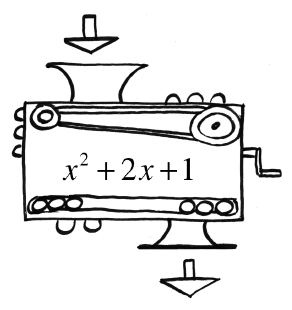Home > A2C > Chapter 1 > Lesson 1.1.2 > Problem1-18

1-18.

Carmichael made a function machine. The inner workings of the machine are visible in the diagram below. What will the output be in each of the following cases?

1. If $3$ is dropped in?

Substitute $3$ for every $x$ (into $x^2+2x+1$).

$(3)^2+2(3)+1$

$16$

2. If $−4$ is dropped in?

Substitute $(−4)$ for every $x$ in the expression.

3. If $−22.872$ is dropped in?

$\approx478.38$